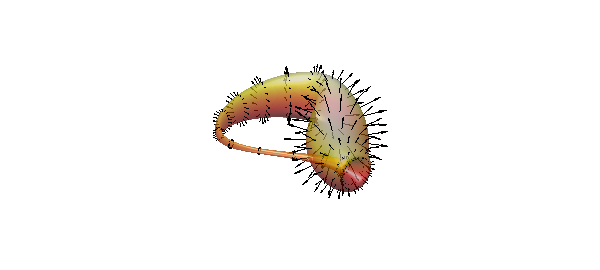This example shows Chebfun2 being used to represent parameterized surfaces. We start by plotting two simple quadratic surfaces that are commonly taught in multivariate calculus: a cone and a hyperboloid of one sheet.

These two surfaces can be parameterized using polar coordinates. We first define the radial and angular parameters as Chebfun2 objects.

u = chebfun2(@(u,v) u, [-1 1 0 2*pi]);
v = chebfun2(@(u,v) v, [-1 1 0 2*pi]);

Here we plot a cone.

x = u.*cos(v); y = u.*sin(v); z = u;
surf(x,y,z), axis equal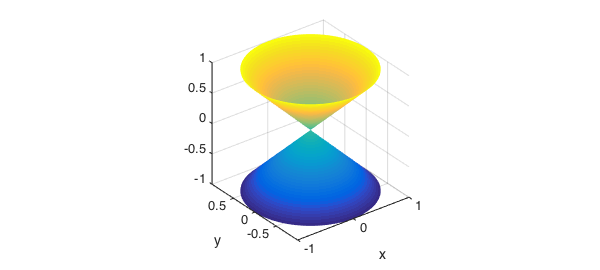And here we graph a hyperboloid of one sheet.

x = sqrt(1/4+u.^2).*cos(v); y = sqrt(1/4+u.^2).*sin(v); z = u;
surf(x,y,z), axis equal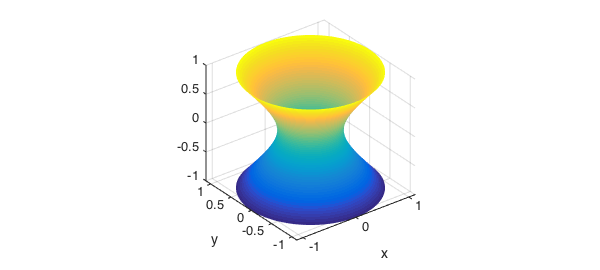We can also define functions over such surfaces. Here is one example:

f = (1-u).*sin(10*v);
surf(x,y,z,f), axis equal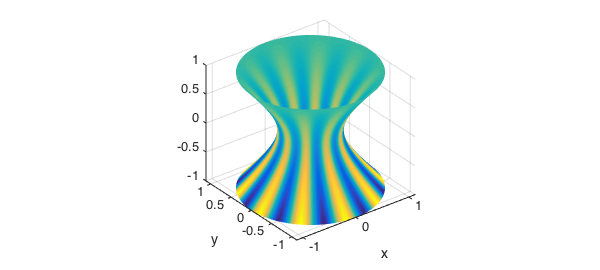We now generate a bumpy sphere. We start with a parameterization based on standard spherical coordinates.

t = chebfun2(@(t,p) t,[0 2*pi -pi/2 pi/2]);
p = chebfun2(@(t,p) p,[0 2*pi -pi/2 pi/2]);
[x,y,z] = sph2cart(t,p,0*t+1);
surf(x,y,z), axis equal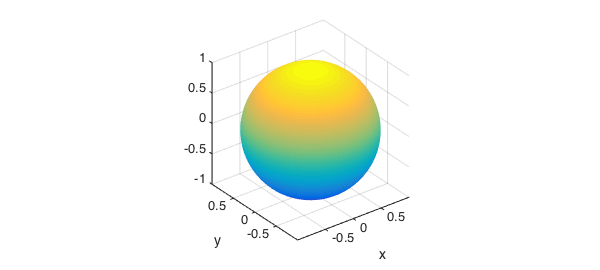To make it bumpy, we perturb its radius with an oscillatory function.

delta = sin(20*x)+sin(20*y)+sin(20*z);
[x,y,z] = sph2cart(t,p,1+0.05*delta);
surf(x,y,z), axis equal tight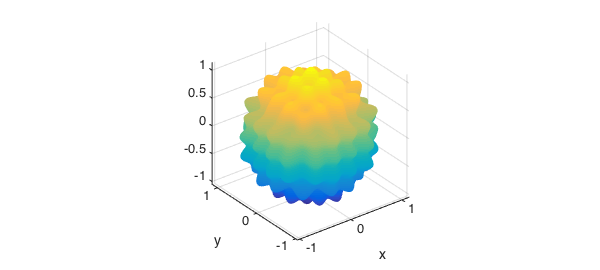Finally we define a function on its surface.

f = (sin(10*t)).*cos(15*p);
surf(x,y,z,f), axis equal tight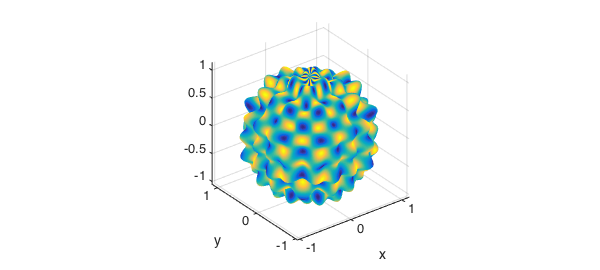Here is the top view:

view(0,90)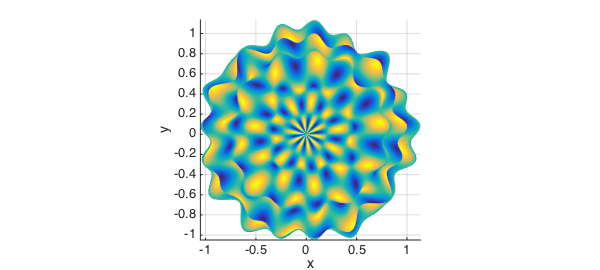There are many other interesting surfaces that can be represented by Chebfun2 objects. The parameterization of this seashell was taken from .

u = chebfun2(@(u,v) u, [0 6*pi 0 2*pi]);
v = chebfun2(@(u,v) v, [0 6*pi 0 2*pi]);
x = 2*(1-exp(u/(6*pi))).*cos(u).*cos(v/2).^2;
y = 2*(-1+exp(u/(6*pi))).*sin(u).*cos(v/2).^2;
z = 1-exp(u/(3*pi))-sin(v)+exp(u/(6*pi)).*sin(v);
surf(x,y,z), camlight
view(160,10), axis equal, box on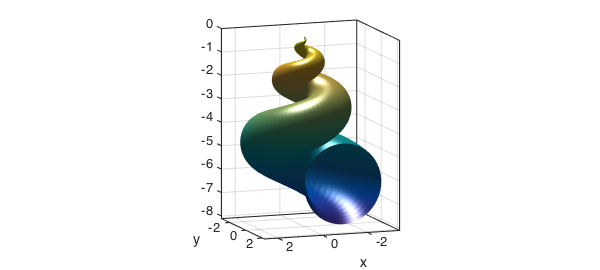Here is the top view:

view(-180,90)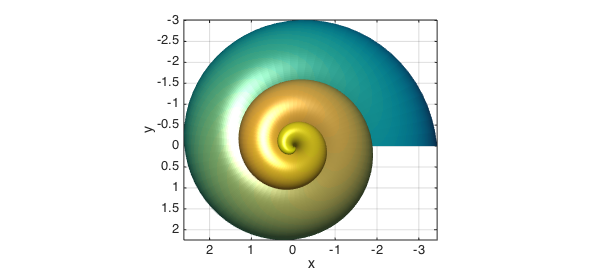And here is the well known Moebius strip, see , often used as an example of a non-orientable surface.

u = chebfun2(@(u,v) u, [0 2*pi -1 1]);
v = chebfun2(@(u,v) v, [0 2*pi -1 1]);
x = (1+0.5*v.*cos(u/2)).*cos(u);
y = (1+0.5*v.*cos(u/2)).*sin(u);
z = 0.5*v.*sin(u/2);
surf(x,y,z), camlight, axis equal, box on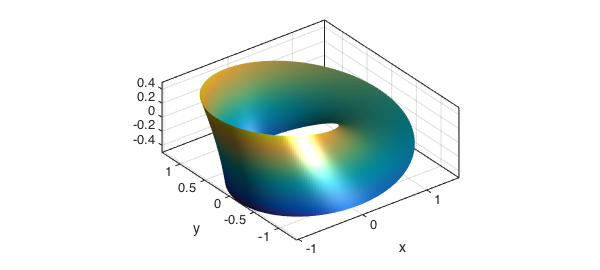What happens if we try to plot normal vectors to this surface? We can compute a normal vector field to this surface using the command NORMAL, which take a position vector as input.

r = [x;y;z];
n = normal(r);
hold on,
quiver3(x,y,z,n,2,'k','numpts',8)
axis tight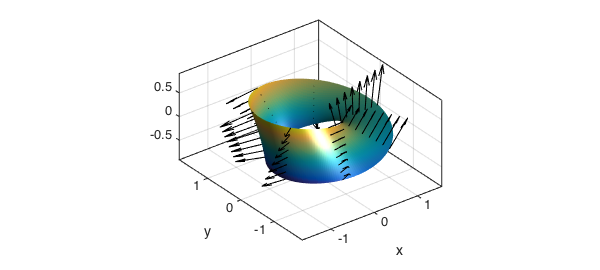The normal, as computed, is clearly not continuous on this surface. The normal vector field computed above is $ru$ x $rv$. Both $ru$ and $rv$ are parallel to the surface. We illustrate this below

ru = diff(r,1,1);
rv = diff(r,1,2);
quiver3(x,y,z,ru,'r','numpts',8)
quiver3(x,y,z,rv,'b','numpts',8)
view(-80,65);
hold off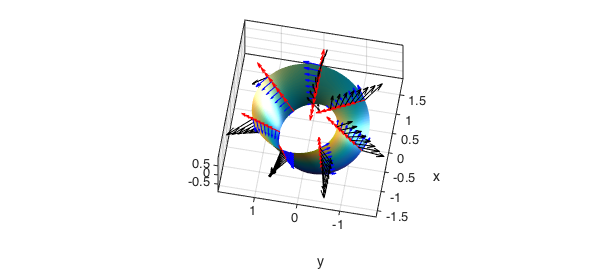We can verify that $ru$ and $rv$ are orthogonal, in this case, by taking their inner product.

norm(ru'*rv,inf)
ans =
5.016985192747734e-14


Finally, we take advantage of this orthogonality and project an arbitrary vector field $V$ onto the Moebius surface. In the figure below, the field PV is tangent to the surface.

R1 = ru/sqrt(ru'*ru);
R2 = rv/sqrt(rv'*rv);
V = [sin(5*u);cos(5*v);0];
PV = (R1'*V)*R1 + (R2'*V)*R2;
surf(x,y,z), axis tight, view(-80,65), camlight
hold on
quiver3(x,y,z,PV,2,'k','numpts',30)
hold off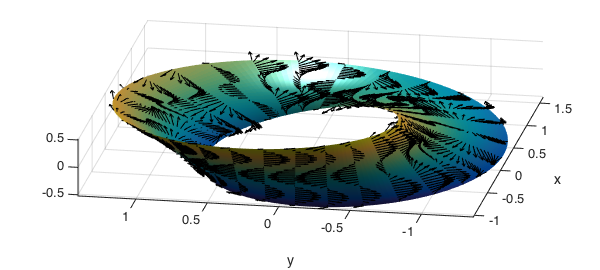Our final example is the Klein Bottle, see , which is also non-orientable. A plot of the "figure 8" immersion (Klein bagel) of the Klein bottle is below.

u = chebfun2(@(u,v) u, [0 2*pi 0 2*pi]);
v = chebfun2(@(u,v) v, [0 2*pi 0 2*pi]);
r = 3;
x=(r+cos(u/2).*sin(v)-sin(u/2).*sin(2*v)).*cos(u);
y=(r+cos(u/2).*sin(v)-sin(u/2).*sin(2*v)).*sin(u);
z=sin(u/2).*sin(v)+cos(u/2).*sin(2*v);
surf(x,y,z,'FaceAlpha',.6), camlight left, colormap(hot), axis tight equal off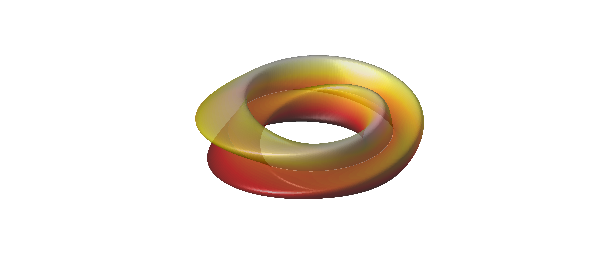And here are the top and side views.

view(0,90)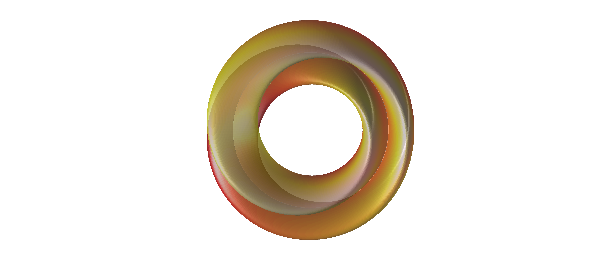view(90,0)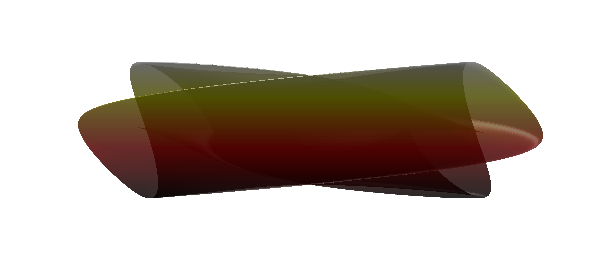Here is the more complicated version found by Robert Israel of the parameterization of the 3-dimensional immersion of the bottle itself.

u = chebfun2(@(u,v) u, [0 pi 0 2*pi]);
v = chebfun2(@(u,v) v, [0 pi 0 2*pi]);
x = -(2/15)*cos(u).*(3*cos(v)-30*sin(u)+90*cos(u).^4.*sin(u)- ...
60*cos(u).^6.*sin(u)+5*cos(u).*cos(v).*sin(u));
y = -(1/15)*sin(u).*(3*cos(v)-3*cos(u).^2.*cos(v)-48*cos(u).^4.*cos(v)+ ...
48*cos(u).^6.*cos(v)-60*sin(u)+5*cos(u).*cos(v).*sin(u) ...
-5*cos(u).^3.*cos(v).*sin(u) -80*cos(u).^5.*cos(v).*sin(u)+ ...
80*cos(u).^7.*cos(v).*sin(u));
z = (2/15)*(3+5*cos(u).*sin(u)).*sin(v);
surf(x,y,z,'FaceAlpha',.5), camlight left, axis tight equal off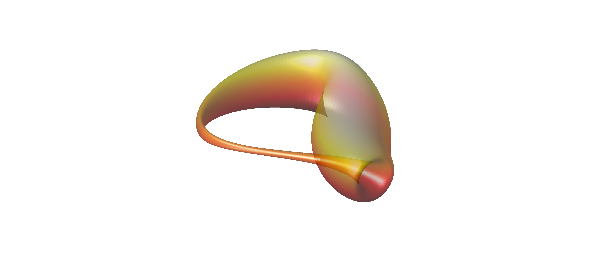A normal vector field on this surface can now be obtained using Chebfun2 with a simple command.

hold on
quiver3(x,y,z,-normal([x;y;z]),2,'k')
hold off